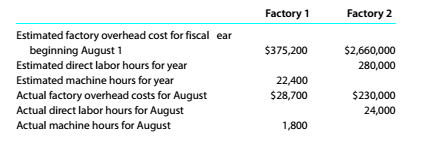Chapter 10, Problem 10.14E

Chapter
Section
Textbook Problem

Factory overhead rates and account balances Prostheses Industries operates two factories. The manufacturing operations of Factory 1 are machine intensive, while the manufacturing operations of Factory 2 are labor intensive. The company applies factory overhead to jobs on the basis of machine hours in Factory I and on the basis of direct labor hours in Factory 2. Estimated factory overhead costs, direct labor hours, and machine hours are as follows:a. Determine the factory overhead rate for Factory 1. b.Determine the factory overhead rate for Factory 2. c. Determine the factory overhead applied to production in each factory for January. d.Determine the balances of the factory accounts for each factory as of January 31, and indicate whether the amounts represent overapplied or underapplied factory overiiead. e.Explain why Factory 1 uses machine hours to allocate factory overhead while Factory 2 uses direct labor hours.

To determine

(a)

Concept Introduction:

Factory overhead rate means the rate which is calculated as per the basis given for allocating the overhead expenses related to factory overhead incurred in manufacturing process.

To Compute:

The Factory overhead rate for factory 1.

Explanation

Computation of factory overhead rate for factory 1 is follows:

 Particulars Factory 1 Estimated factory overhead cost for fiscal year (A) \$375,20
To determine

(b)

Concept Introduction:

Factory overhead rate means the rate which is calculated as per the basis given for allocating the overhead expenses related to factory overhead incurred in manufacturing process.

To compute:

The Factory overhead rate for factory 2.

To determine

(c)

Concept Introduction:

Factory overhead rate means the rate which is calculated as per the basis given for allocating the overhead expenses related to factory overhead incurred in manufacturing process.

To compute:

The factory overhead applied to production in each factory.

To determine

(d)

Concept Introduction:

Factory overhead rate means the rate which is calculated as per the basis given for allocating the overhead expenses related to factory overhead incurred in manufacturing process.

To compute:

The balances of the factory account for each factory and also explain whether the factory overhead is over applied or under applied.

To determine

(e)

Concept Introduction:

Factory overhead rate means the rate which is calculated as per the basis given for allocating the overhead expenses related to factory overhead incurred in manufacturing process.

To explain:

The reason of using machine hours by factory 1 and direct labor hours by factory 2 for allocating factory overhead.

Still sussing out bartleby?

Check out a sample textbook solution.

See a sample solution

The Solution to Your Study Problems

Bartleby provides explanations to thousands of textbook problems written by our experts, many with advanced degrees!

Get Started

Find more solutions based on key concepts